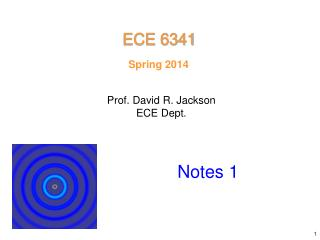DownloadDownload PresentationSpring 2014

# Spring 2014

Download Presentation## Spring 2014

- - - - - - - - - - - - - - - - - - - - - - - - - - - E N D - - - - - - - - - - - - - - - - - - - - - - - - - - -
##### Presentation Transcript

1. ECE 6341 Spring 2014 Prof. David R. Jackson ECE Dept. Notes 1

2. Fields in a Source Free Region Source-free homogeneous region Sources Note: For a lossy region, we replace

3. Fields in a Source Free Region (cont.) (b) (a) Ampere’s law: Faraday’s law: The field can be represented using eitherA or F

4. Fields in a Source Free Region (cont.) Case (a) Assume we use A: This is the “mixed potential” for form the electric field. Hence

5. Fields in a Source Free Region (cont.) (Lorenz Gauge) Choose This is the “vector Helmholtz equation” for the magnetic vector potential. Then

6. Fields in a Source Free Region (cont.) Case (b) Invoking duality: Assume we use F: Choose We then have:

7. Fields in a Source Free Region (cont.) To be even more general, let where (Ea, Ha) and (Ef, Hf) each satisfy Maxwell’s equations. (This is an arbitrary partition.) The representation is not unique, since there are many ways to split the field. For example, we could use

8. Fields in a Source Free Region (cont.) We construct the vector potentials so that We then have

9. Fields in a Source Free Region (cont.) Depending on the type of source (outside the source-free region) that is producing the field within the source-free region, it may be more convenient to represent the field using only A or only F. EXAMPLES Electric dipole: choose only A(A is in the same direction as J) Magnetic dipole: choose only F (F is in the same direction as M) This solution was discussed in ECE 6340. In principle, one could represent the field of the electric dipole using the F vector potential, but it would be much more difficult than with the A vector potential!

10. TEz/TMz Theorem In a source-free homogeneous region, we can always represent the field using the following form: is an arbitraryfixed direction (called here the “pilot vector” direction). (A proof of this theorem is given later.) This theorem gives us a systematic way to represent the fields in a source-free region, involving only two scalar field components.

11. TEz/TMz Theorem (cont.) Note: A simpler solution often results if we choose the best direction for the pilot vector. EXAMPLE An infinitesimal unit-amplitude electric dipole pointing in the z direction: We only need Az and not Fz. The solution is If we had picked the pilot vector direction to be something different, such as x, we would need BOTH Ax and Fx.

12. TEz/TMz Theorem (cont.) TEz / TMzproperty of Fields Consider, for example, (AzTMz) Hence, (FzTEz) Similarly,

13. TEz/TMz Theorem (cont.) The fields are found as follows: Note: There is a factor  difference with the Harrington text.

14. TEz/TMz Theorem (cont.) Note: There is a factor  difference with the Harrington text.

15. TEz/TMz Theorem (cont.) From the vector Helmholtz equation we have: Taking the z component gives: or Similarly, Hence, any EM problem in a source-free region reduces to solving the scalar Helmholtz equation:

16. Summary of TEz/TMzResult Source-free homogeneous region Sources Arbitrary pilot direction “z”

17. Proof of TEz/TMz Theorem S Source-free region Apply equivalence principle: Keep original material and fields inside S. Put zero fields outside S. Put(e,m) outside S.

18. Proof of TEz/TMz Theorem S Infinite homogenous space

19. Proof of TEz/TMz Theorem (cont.) Consider the z component of the currents: (from 6340) Also, from a horizontal dipole source, we can show that: This will be established later in the class notes and the homework by solving the problem of a horizontal (x or y directed) dipole source using Az and Fz. Hence, the fields in the source-free region due to all of the equivalent currents may be represented with Az and Fz.

20. Non-Uniqueness of Potentials AzandFzare not unique. To illustrate, consider: This set of potentials produces a null field! Hence, adding this set of potentials to a solutiondoes not change the fields.